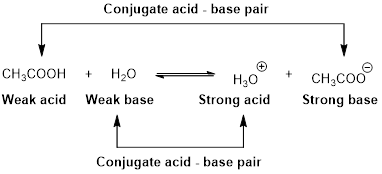Chapter 16, Problem 18PS

Chapter
Section
Textbook Problem

Several acids are listed here with their respective equilibrium constants:HF(aq) + H2O(ℓ) ⇄ H3O+(aq) + F−(aq) Ka = 7.2 × 10−4HPO42−(aq) + H2O(ℓ) ⇄ H3O+(aq) + PO43−(aq) Ka = 3.6 × 10−13CH3CO2(H) + H2O(ℓ) ⇄ H3O+(aq) + CH3CO2−(aq) Ka = 1.8 × 10−5 (a) Which is the strongest acid? Which is the weakest acid? (b) What is the conjugate base of the acid I IF? (c) Which acid has the weakest conjugate base? (d) Which acid has the strongest conjugate base?

(a)

Interpretation Introduction

Interpretation: The strongest acid and the weakest acid has to be identified.

Concept introduction:

Strong acids dissociate completely in water which produce H+ ion, while weak acids dissociate partially in water and produce H+ ion.

An equilibrium constant (K) is the ratio of concentration of products and reactants raised to appropriate stoichiometric coefficient at equlibrium.

For the general acid HA,

HA(aq)+H2O(l)H3O+(aq)+A(aq)

The relative strength of an acid and base in water can be also expressed quantitatively with an equilibrium constant as follows:

Ka=[H3O+][A][HA]

An equilibrium constant (K) with subscript a indicate that it is an equilibrium constant of an acid in water.

Explanation

For the hydrogen flouride (HF),

HF(aq)+H2O(l)H3O+(aq)+F(aq)

Ka=[H3O+][F][HF]

A large value of Ka=7.2×104 indicates that the ionization product is strongly favoured because the product [H3O+][F] is more than the equilibrium concentration of the strong acid [HF].

For the hydrogen phosphate (HPO42),

HPO42(aq)+H2O(l

(b)

Interpretation Introduction

Interpretation: The conjucate base of HF has to be identified.

Concept introduction:

Bronsted –Lowry conjugated acid-Base pairs:

When an acid is dissolved in water, the acid (HA) donates a proton to water to form a new acid (conjugated acid) and a new base (conjugated base).The pair of an Acid –Base differs by a proton called conjugated Acid-Base pair.

(c)

Interpretation Introduction

Interpretation: The weakest conjucate base has to be identified.

Concept introduction:

Bronsted –Lowry conjugated acid-Base pairs:

When an acid is dissolved in water, the acid (HA) donates a proton to water to form a new acid (conjugated acid) and a new base (conjugated base).The pair of an Acid –Base differs by a proton called conjugated Acid-Base pair.

An equilibrium constant (K) is the ratio of concentration of products and reactants raised to appropriate stoichiometric coefficient at equlibrium.

For the general acid HA,

HA(aq)+H2O(l)H3O+(aq)+A(aq)

The relative strength of an acid and base in water can be also expressed quantitatively with an equilibrium constant as follows:

Ka=[H3O+][A][HA]

An equilibrium constant (K) with subscript a indicate that it is an equilibrium constant of an acid in water.

(d)

Interpretation Introduction

Interpretation: The strongest conjucate base has to be identified.

Concept introduction:

Bronsted –Lowry conjugated acid-Base pairs:

When an acid is dissolved in water, the acid (HA) donates a proton to water to form a new acid (conjugated acid) and a new base (conjugated base).The pair of an Acid –Base differs by a proton called conjugated Acid-Base pair.

An equilibrium constant (K) is the ratio of concentration of products and reactants raised to appropriate stoichiometric coefficient at equlibrium.

For the general acid HA,

HA(aq)+H2O(l)H3O+(aq)+A(aq)

The relative strength of an acid and base in water can be also expressed quantitatively with an equilibrium constant as follows:

Ka=[H3O+][A][HA]

An equilibrium constant (K) with subscript a indicate that it is an equilibrium constant of an acid in water.

Still sussing out bartleby?

Check out a sample textbook solution.

See a sample solution

The Solution to Your Study Problems

Bartleby provides explanations to thousands of textbook problems written by our experts, many with advanced degrees!

Get Started

Find more solutions based on key concepts